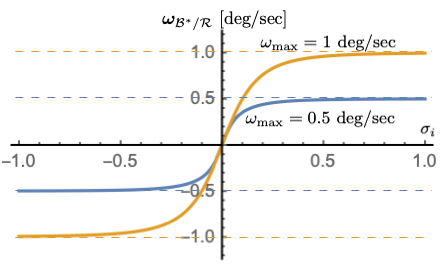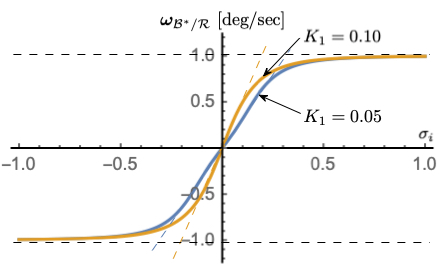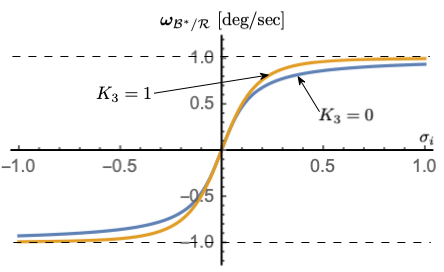# Module: mrpSteering¶

## Executive Summary¶

The intend of this module is to implement an MRP attitude steering law where the control output is a vector of commanded body rates. To use this module it is required to use a separate rate tracking servo control module, such as Module: rateServoFullNonlinear, as well.

## Message Connection Descriptions¶

The following table lists all the module input and output messages. The module msg connection is set by the user from python. The msg type contains a link to the message structure definition, while the description provides information on what this message is used for.Figure 1: mrpSteering() Module I/O Illustration

Module I/O Messages

Msg Variable Name

Msg Type

Description

guidInMsg

Attitude guidance input message.

rateCmdOutMsg

Rate command output message.

## Detailed Module Description¶

The following text describes the mathematics behind the mrpSteering module. Further information can also be found in the journal paper Speed-Constrained Three-Axes Attitude Control Using Kinematic Steering.

### Steering Law Goals¶

This technical note develops a new MRP based steering law that drives a body frame $${\cal B}:\{ \hat{\bf b}_1, \hat{\bf b}_2, \hat{\bf b}_3 \}$$ towards a time varying reference frame $${\cal R}:\{ \hat{\bf r}_1, \hat{\bf r}_2, \hat{\bf r}_3 \}$$. The inertial frame is given by $${\cal N}:\{ \hat{\bf n}_1, \hat{\bf n}_2, \hat{\bf n}_3 \}$$. The RW coordinate frame is given by $$\mathcal{W}_{i}:\{ \hat{\bf g}_{s_{i}}, \hat{\bf g}_{t_{i}}, \hat{\bf g}_{g_{i}} \}$$. Using MRPs, the overall control goal is

(1)$\pmb\sigma_{\mathcal{B}/\mathcal{R}} \rightarrow 0$

The reference frame orientation $$\pmb \sigma_{\mathcal{R}/\mathcal{N}}$$, angular velocity $$\pmb\omega_{\mathcal{R}/\mathcal{N}}$$ and inertial angular acceleration $$\dot{\pmb \omega}_{\mathcal{R}/\mathcal{N}}$$ are assumed to be known.

The rotational equations of motion of a rigid spacecraft with N Reaction Wheels (RWs) attached are given by Analytical Mechanics of Space Systems.

(2)$[I_{RW}] \dot{\pmb \omega} = - [\tilde{\pmb \omega}] \left( [I_{RW}] \pmb\omega + [G_{s}] \pmb h_{s} \right) - [G_{s}] {\bf u}_{s} + {\bf L}$

where the inertia tensor $$[I_{RW}]$$ is defined as

(3)$[I_{RW}] = [I_{s}] + \sum_{i=1}^{N} \left (J_{t_{i}} \hat{\bf g}_{t_{i}} \hat{\bf g}_{t_{i}}^{T} + J_{g_{i}} \hat{\bf g}_{g_{i}} \hat{\bf g}_{g_{i}}^{T} \right)$

The spacecraft inertial without the N RWs is $$[I_{s}]$$, while $$J_{s_{i}}$$, $$J_{t_{i}}$$ and $$J_{g_{i}}$$ are the RW inertias about the body fixed RW axis $$\hat{\bf g}_{s_{i}}$$ (RW spin axis), $$\hat{\bf g}_{t_{i}}$$ and $$\hat{\bf g}_{g_{i}}$$. The $$3\times N$$ projection matrix $$[G_{s}]$$ is then defined as

(4)$[G_{s}] = \begin{bmatrix} \cdots {}^{B}{\hat{\bf g}}_{s_{i}} \cdots \end{bmatrix}$

The RW inertial angular momentum vector $${\bf h}_{s}$$ is defined as

(5)$h_{s_{i}} = J_{s_{i}} (\omega_{s_{i}} + \Omega_{i})$

Here $$\Omega_{i}$$ is the $$i^{\text{th}}$$ RW spin relative to the spacecraft, and the body angular velocity is written in terms of body and RW frame components as

(6)$\pmb\omega = \omega_{1} \hat{\bf b}_{1} + \omega_{2} \hat{\bf b}_{2} + \omega_{3} \hat{\bf b}_{3} = \omega_{s_{i}} \hat{\bf g}_{s_{i}} + \omega_{t_{i}} \hat{\bf g}_{t_{i}} + \omega_{g_{i}} \hat{\bf g}_{g_{i}}$

### MRP Steering Law¶

#### Steering Law Stability Requirement¶

As is commonly done in robotic applications where the steering laws are of the form $$\dot{\bf x} = {\bf u}$$, this section derives a kinematic based attitude steering law. Let us consider the simple Lyapunov candidate function:

(7)$V ( \pmb\sigma_{\mathcal{B}/\mathcal{R}} ) = 2 \ln \left ( 1 + \pmb\sigma_{\mathcal{B}/\mathcal{R}} ^{T} \pmb\sigma_{\mathcal{B}/\mathcal{R}} \right)$

in terms of the MRP attitude tracking error $$\pmb\sigma_{\mathcal{B}/\mathcal{R}}$$. Using the MRP differential kinematic equations

(8)$\begin{split} \dot{\pmb\sigma}_{\mathcal{B}/\mathcal{R}} &= \frac{1}{4}[B(\pmb\sigma_{\mathcal{B}/\mathcal{R}})] {}^{B}{\pmb\omega}_{\mathcal{B}/\mathcal{R}} \\ &= \frac{1}{4} \left[ (1-\sigma_{\mathcal{B}/\mathcal{R}}^{2})[I_{3\times 3} + 2 [\tilde{\pmb\sigma}_{\mathcal{B}/\mathcal{R}}] + 2 \pmb\sigma_{\mathcal{B}/\mathcal{R}} \pmb\sigma_{\mathcal{B}/\mathcal{R}}^{T} \right] {}^{B}{\pmb\omega}_{\mathcal{B}/\mathcal{R}}\end{split}$

where $$\sigma_{\mathcal{B}/\mathcal{R}}^{2} = \pmb\sigma_{\mathcal{B}/\mathcal{R}}^{T} \pmb\sigma_{\mathcal{B}/\mathcal{R}}$$, the time derivative of $$V$$ is

(9)$\dot V =\pmb\sigma_{\mathcal{B}/\mathcal{R}}^{T} \left( {}^{B}{ \pmb\omega}_{\mathcal{B}/\mathcal{R}} \right)$

To create a kinematic steering law, let $${\mathcal{B}}^{\ast}$$ be the desired body orientation, and $$\pmb\omega_{{\mathcal{B}}^{\ast}/\mathcal{R}}$$ be the desired angular velocity vector of this body orientation relative to the reference frame $$\mathcal{R}$$. The steering law requires an algorithm for the desired body rates $$\pmb\omega_{{\mathcal{B}}^{\ast}/\mathcal{R}}$$ relative to the reference frame make $$\dot V$$ in Eq. (9) negative definite. For this purpose, let us select

(10)${}^{B}{\pmb\omega}_{{\mathcal{B}}^{\ast}/\mathcal{R}} = - {\bf f}(\pmb\sigma_{\mathcal{B}/\mathcal{R}})$

where $${\bf f}(\pmb\sigma)$$ is an even function such that

(11)$\pmb\sigma ^{T} {\bf f}(\pmb\sigma) > 0$

The Lyapunov rate simplifies to the negative definite expression:

(12)$\dot V = - \pmb\sigma_{\mathcal{B}/\mathcal{R}}^{T} {\bf f}(\pmb\sigma_{\mathcal{B}/\mathcal{R}}) < 0$

#### Saturated MRP Steering Law¶

A very simple example would be to set

(13)${\bf f} (\pmb\sigma_{\mathcal{B}/\mathcal{R}}) = K_{1} \pmb\sigma_{\mathcal{B}/\mathcal{R}}$

where $$K_{1}>0$$. This yields a kinematic control where the desired body rates are proportional to the MRP attitude error measure. If the rate should saturate, then $${\bf f}()$$ could be defined as

(14)$\begin{split} {\bf f}(\pmb\sigma_{\mathcal{B}/\mathcal{R}}) = \begin{cases} K_{1} \sigma_{i} &\text{if } |K_{1} \sigma_{i}| \le \omega_{\text{max}} \\ \omega_{\text{max}} \text{sgn}(\sigma_{i}) &\text{if } |K_{1} \sigma_{i}| > \omega_{\text{max}} \end{cases}\end{split}$

where

$\pmb\sigma_{\mathcal{B}/\mathcal{R}} = (\sigma_{1}, \sigma_{2}, \sigma_{3})^{T}$

A smoothly saturating function is given by

(15)${\bf f}(\pmb\sigma_{\mathcal{B}/\mathcal{R}}) = \arctan \left( \pmb\sigma_{\mathcal{B}/\mathcal{R}} \frac{K_{1} \pi}{2 \omega_{\text{max}}} \right) \frac{2 \omega_{\text{max}}}{\pi}$

where

(16)$\begin{split} {\bf f}(\pmb\sigma_{\mathcal{B}/\mathcal{R}}) = \begin{pmatrix} f(\sigma_{1})\\ f(\sigma_{2})\\ f(\sigma_{3}) \end{pmatrix}\end{split}$

Here as $$\sigma_{i} \rightarrow \infty$$ then the function $$f$$ smoothly converges to the maximum speed rate $$\pm \omega_{\text{max}}$$. For small $$|\pmb\sigma_{\mathcal{B}/\mathcal{R}}|$$, this function linearizes to

${\bf f}(\pmb\sigma_{\mathcal{B}/\mathcal{R}}) \approx K_{1} \pmb\sigma_{\mathcal{B}/\mathcal{R}} + \text{ H.O.T}$

If the MRP shadow set parameters are used to avoid the MRP singularity at 360 deg, then $$|\pmb\sigma_{\mathcal{B}/\mathcal{R}}|$$ is upper limited by 1. To control how rapidly the rate commands approach the $$\omega_{\text{max}}$$ limit, Eq. (15) is modified to include a cubic term:

(17)$f( \sigma_{i}) = \arctan \left( (K_{1} \sigma_{i} +K_{3} \sigma_{i}^{3}) \frac{ \pi}{2 \omega_{\text{max}}} \right) \frac{2 \omega_{\text{max}}}{\pi}$

The order of the polynomial must be odd to keep ${bf f}()$ an even function. A nice feature of Eq. (17) is that the control rate is saturated individually about each axis. If the smoothing component is removed to reduce this to a bang-band rate control, then this would yield a Lyapunov optimal control which minimizes $$\dot V$$ subject to the allowable rate constraint $$\omega_{\text{max}}$$.Figure 2: $$\omega_{\text{max}}$$ dependency with $$K_{1} = 0.1$$, $$K_{3} = 1$$Figure 3: $$K_{1}$$ dependency with $$\omega_{\text{max}}$$ = 1 deg/s, $$K_{3} = 1$$Figure 4: $$K_{3}$$ dependency with $$\omega_{\text{max}}$$ = 1 deg/s, $$K_{1} = 0.1$$

Figures 2-4 illustrate how the parameters $$\omega_{\text{max}}$$, $$K_{1}$$ and $$K_{3}$$ impact the steering law behavior. The maximum steering law rate commands are easily set through the $$\omega_{\text{max}}$$ parameters. The gain $$K_{1}$$ controls the linear stiffness when the attitude errors have become small, while $$K_{3}$$ controls how rapidly the steering law approaches the speed command limit.

The required velocity servo loop design is aided by knowing the body-frame derivative of $${}^{B}{\pmb\omega}_{{\mathcal{B}}^{\ast}/\mathcal{R}}$$ to implement a feed-forward components. Using the $${\bf f}()$$ function definition in Eq. (16), this requires the time derivatives of $$f(\sigma_{i})$$.

$\begin{split}\frac{{}^{B}{\text{d} ({}^{B}{\pmb\omega}_{{\mathcal{B}}^{\ast}/\mathcal{R}} ) }}{\text{d} t} = {\pmb\omega}_{{\mathcal{B}}^{\ast}/\mathcal{R}} ' = - \frac{\partial {\bf f}}{\partial \pmb\sigma_{{\mathcal{B}}^{\ast}/\mathcal{R}}} \dot{\pmb\sigma}_{{\mathcal{B}}^{\ast}/\mathcal{R}} = - \begin{pmatrix} \frac{\partial f}{\partial \sigma_{1}} \dot{ \sigma}_{1} \\ \frac{\partial f}{\partial \sigma_{2}} \dot{ \sigma}_{2} \\ \frac{\partial f}{\partial \sigma_{3}} \dot{ \sigma}_{3} \end{pmatrix}\end{split}$

where

$\begin{split}\dot{\pmb\sigma} _{{\mathcal{B}}^{\ast}/\mathcal{R}} = \begin{pmatrix} \dot\sigma_{1}\\ \dot\sigma_{2}\\ \dot\sigma_{3} \end{pmatrix} = \frac{1}{4}[B(\pmb\sigma_{{\mathcal{B}}^{\ast}/\mathcal{R}})] {}^{B}{\pmb\omega}_{{\mathcal{B}}^{\ast}/\mathcal{R}}\end{split}$

Using the general $$f()$$ definition in Eq. (17), its sensitivity with respect to $$\sigma_{i}$$ is

$\frac{ \partial f }{ \partial \sigma_{i} } = \frac{ (K_{1} + 3 K_{3} \sigma_{i}^{2}) }{ 1+(K_{1}\sigma_{i} + K_{3} \sigma_{i}^{3})^{2} \left(\frac{\pi}{2 \omega_{\text{max}}}\right)^{2} }$

## Module Assumptions and Limitations¶

This control assumes the spacecraft is rigid, and that a fast enough rate control sub-servo system is present.

## User Guide¶

The following variables must be specified from Python:

• The gains K1, K3

• The value of omega_max

This module returns the values of $$\pmb\omega_{\mathcal{B}^{\ast}/\mathcal{R}}$$ and $$\pmb\omega_{\mathcal{B}^{\ast}/\mathcal{R}}'$$, which are used in the rate servo-level controller to compute required torques.

The control update period $$\Delta t$$ is evaluated automatically.

Functions

void SelfInit_mrpSteering(mrpSteeringConfig *configData, int64_t moduleID)

self init method

Parameters
• configData – The configuration data associated with this module

• moduleID – The module identifier

Returns

void

void Update_mrpSteering(mrpSteeringConfig *configData, uint64_t callTime, int64_t moduleID)

This method takes the attitude and rate errors relative to the Reference frame, as well as the reference frame angular rates and acceleration

Parameters
• configData – The configuration data associated with the MRP Steering attitude control

• callTime – The clock time at which the function was called (nanoseconds)

• moduleID – The module identifier

Returns

void

void Reset_mrpSteering(mrpSteeringConfig *configData, uint64_t callTime, int64_t moduleID)

This method performs a complete reset of the module. Local module variables that retain time varying states between function calls are reset to their default values.

Parameters
• configData – The configuration data associated with the MRP steering control

• callTime – The clock time at which the function was called (nanoseconds)

• moduleID – The module identifier

Returns

void

void MRPSteeringLaw(mrpSteeringConfig *configData, double sigma_BR, double omega_ast, double omega_ast_p)

This method computes the MRP Steering law. A commanded body rate is returned given the MRP attitude error measure of the body relative to a reference frame. The function returns the commanded body rate, as well as the body frame derivative of this rate command.

Parameters
• configData – The configuration data associated with this module

• sigma_BR – MRP attitude error of B relative to R

• omega_ast – Commanded body rates

• omega_ast_p – Body frame derivative of the commanded body rates

Returns

void

struct mrpSteeringConfig
#include <mrpSteering.h>

Data structure for the MRP feedback attitude control routine.

Public Members

double K1

[rad/sec] Proportional gain applied to MRP errors

double K3

[rad/sec] Cubic gain applied to MRP error in steering saturation function

double omega_max

[rad/sec] Maximum rate command of steering control

uint32_t ignoreOuterLoopFeedforward

[] Boolean flag indicating if outer feedforward term should be included

RateCmdMsg_C rateCmdOutMsg

rate command output message

AttGuidMsg_C guidInMsg

attitude guidance input message

BSKLogger *bskLogger

BSK Logging.